# Six-sided polygon

In a six-sided polygon. The first two angles are equal, the third angle is twice (the equal angles), two other angles are trice the equal angle, while the last angle is a right angle. Find the value of each angle.

Result

A =  63
B =  63
C =  126
D =  189
E =  189
F =  90

#### Solution:

A=B
C = 2B
D = E
D = 3A
F = 90
A+B+C+D+E+F = (6-2) * 180

A-B = 0
2B-C = 0
D-E = 0
3A-D = 0
F = 90
A+B+C+D+E+F = 720

A = 63
B = 63
C = 126
D = 189
E = 189
F = 90

Calculated by our linear equations calculator.

Leave us a comment of example and its solution (i.e. if it is still somewhat unclear...):

Showing 0 comments:Be the first to comment!#### To solve this example are needed these knowledge from mathematics:

Do you have a system of equations and looking for calculator system of linear equations? See also our trigonometric triangle calculator.

## Next similar examples:

1. In a 2In a thirteen sided polygon, the sum of five angles is 1274°, four of the eight angles remaining are equal and the other four are 18° less than each of the equal angles. Find the angles. .
2. Rotatable tower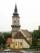Rotatable tower situated in the city center has ground shape of a regular polygon. If the tower is rotated by 14.4° around its centerpiece it looks from the side same. Your task is to calculate at least how many vertices can have a ground plan view of the.
3. Largest angle of the triangleWhat is the largest angle of the triangle if the second angle is 10° greater than twice the first and the third is 30° smaller than the second?
4. Internal anglesFind the internal angles of the triangle ABC if the angle at the vertex C is twice the angle at the B and the angle at the vertex B is 4 degrees smaller than the angle at the vertex A.
5. AnglesThe triangle is one outer angle 158°54' and one internal angle 148°. Calculate the other internal angles of a triangle.
6. AnglesIn the triangle ABC, the ratio of angles is: a:b = 4: 5. The angle c is 36°. How big are the angles a, b?
7. Internal angles IST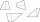Determine internal angles of isosceles trapezium ABCD /a, c are the bases/ and if: alpha:gamma = 1:3
8. Angles of a triangleIn the triangle ABC, the angle beta is 15° greater than the angle alpha. The remaining angle is 30° greater than the sum of the angles alpha and beta. Calculate the angles of a triangle.
9. Triangle 42Triangle BCA. Angles A=119° B=(3y+14) C=4y. What is measure of triangle BCA=?
10. Angles in triangleThe triangle is ratio of the angles β:γ = 6:8. Angle α is 40° greater than β. What are the size of angles of the triangle?
11. Obtuse angleWhich obtuse angle is creating clocks at 17:00?
12. Hexagon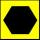Draw a regular hexagon inscribed in a circle with radius r=8 cm. What is its perimeter?
13. Answer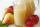Mam used 9 red, 9 green apples and 3 pears to prepare juice. How many pieces of fruit used by mom to prepare this juice?
14. MedianThe median of the triangle LMN is away from vertex N 84 cm. Calculate the length of the median, which start at N.
15. Winnie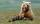Winnie Hugo had the right paw 10 punches and on 15 left more. How many punches has on left paw?
16. Roman numerals 2+Add up the number writtens in Roman numerals. Write the results as a roman numbers.
17. TriangleProve whether you can construct a triangle ABC, if a=9 cm, b=10 cm, c=4 cm.